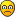## Nonlinear Taylor rule estimation in eviews (HOW TO INCLUDE MONETARY POLICY INERTIA IN MY NONLINEAR EQUATION???!)

For technical questions regarding estimation of single equations, systems, VARs, Factor analysis and State Space Models in EViews. General econometric questions and advice should go in the Econometric Discussions forum.

Moderators: EViews Gareth, EViews Moderator

milami
Posts: 1
Joined: Fri Jun 04, 2021 8:44 am

### Nonlinear Taylor rule estimation in eviews (HOW TO INCLUDE MONETARY POLICY INERTIA IN MY NONLINEAR EQUATION???!)

Hello everybody,

I REALLY NEED HELPI am facing a big problem estimating a nonlinear Dynamic Taylor rule using Smooth Transition Regression (STR) in eviews 11.
As you know, in theory, linear taylor rule takes generally this form: i(t)=ρi(t-1)+(1-ρ)(r^*+α(π(t)-π^* )+β(y(t)-y^* ))+ε(t),
In estimating this linear taylor rule using OLS method, we have the possibility to write in eviews the whole equation that way:
i = c(1)*i(-1) + (1-c(1))*(c(2)*inflation+ c(3)*output_gap)
this possibility gives us the right estimation of monetary policy inertia.

BUT in Non linear model STR, we cannot write the equation explicitly in eviews to estimate it, we therefore have the unique possibility of writing it using dependant variable followed by regressors (ex: i i(-1) inflation output_gap) => this method dosen't give us the possibility to estimate the coefficient ρ (or c(1)) of monetary policy inertia!!
HOW can take into account c(1) and (1-c(1)) in my taylor rule when using an STR model (non linear taylor rule)? is there any trick i can use?

PLEASE HELP MERegards,

Mila.